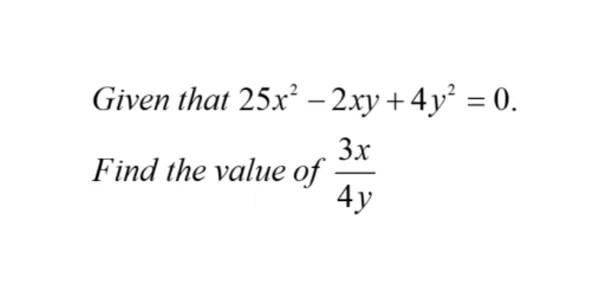e-math-algebraic-equations-find-3x-divide-4y-from-the-algebraic-equation